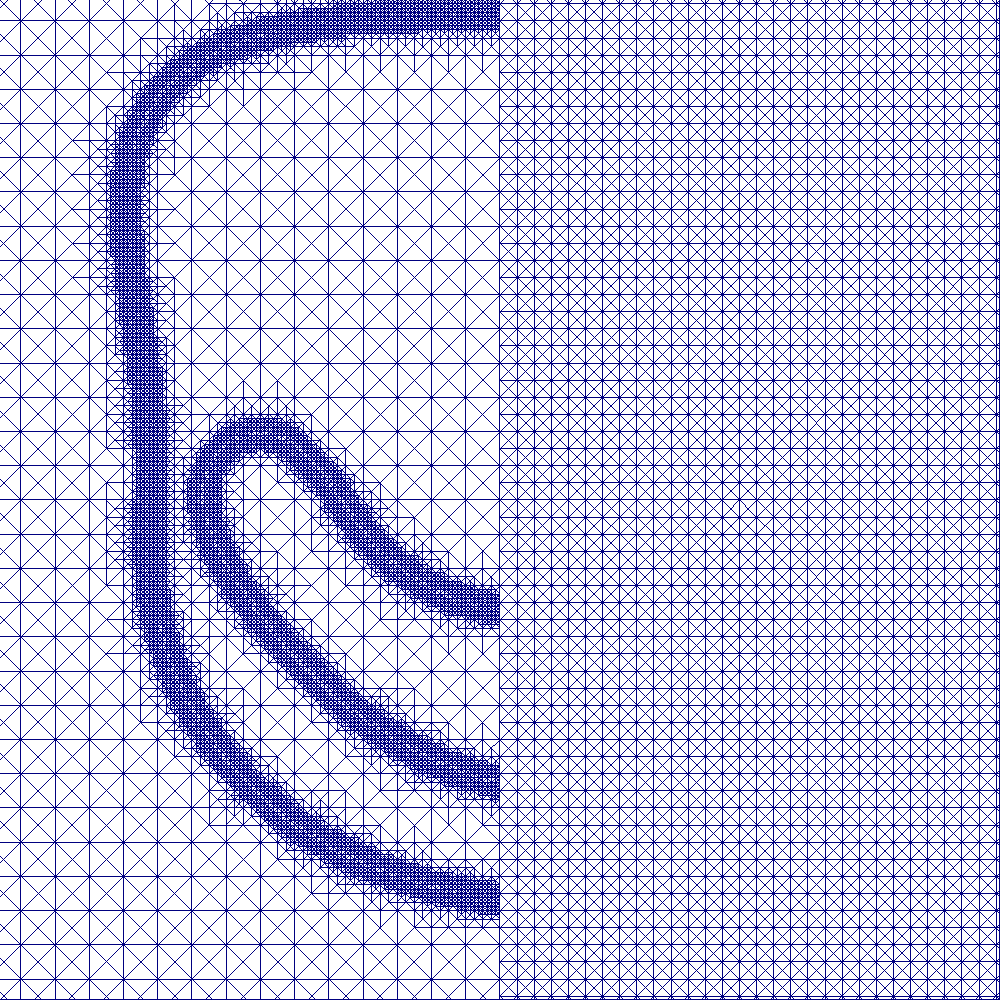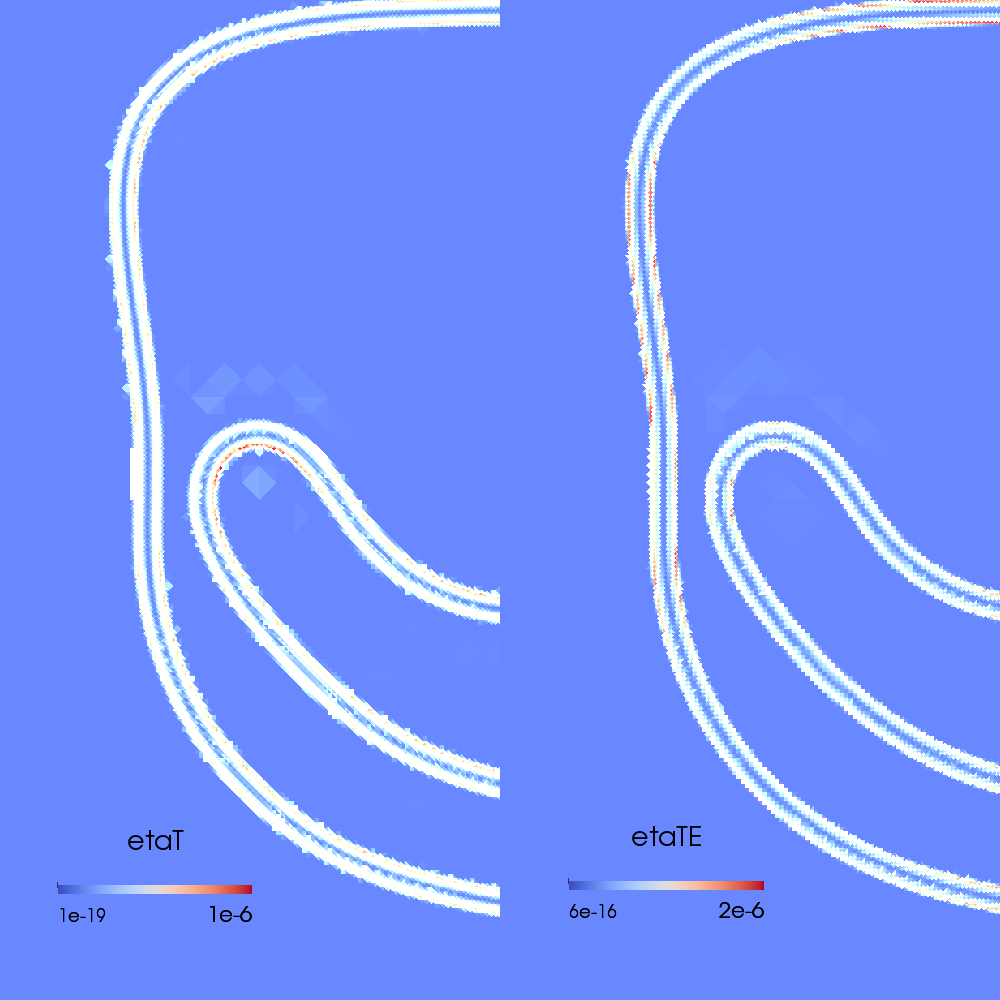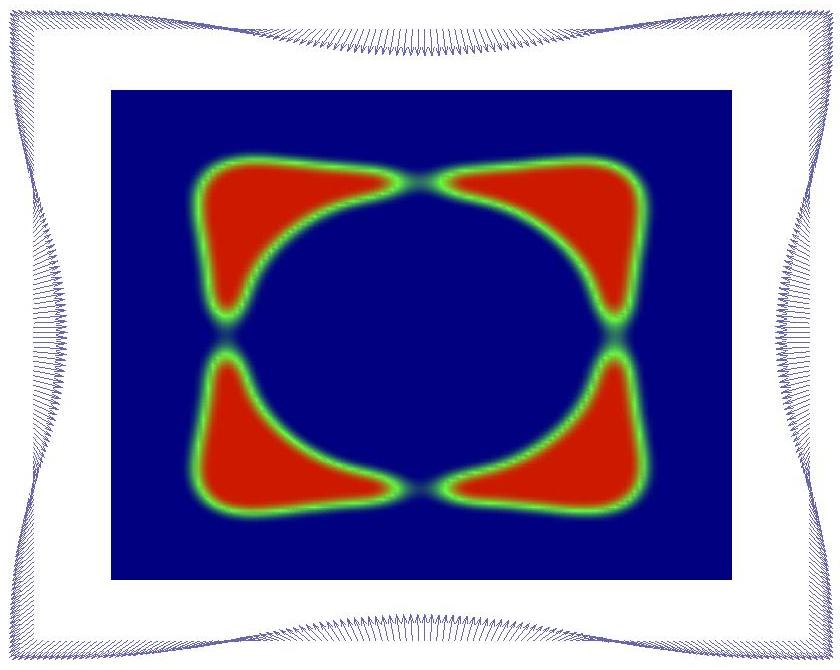# Project Hintermüller / Hinze

Fully adaptive and integrated numerical methods for the simulation and control of variable density multiphase flows governed by diff use interface models

Within this project we develop, analyze, and implement simulation and optimization procedures for variable density multiphase flows governed by di ffuse interface models. In the simulation part we in particular develop and analyse numerical methods for the simulation of multiphase flow problems with variable fluid densities which guarantee a locally re fined resolution of the local processes at the interface. In a next step we propose adaptive discretization concepts for the coupling of diff use interface models and surface partial di fferential equations (PDEs). In the optimization part we consider open - and closed - loop control approaches, where we formulate and analyze optimal control problems for multiphase flows governed by di ffuse interface models, develop robust and reliable solution strategies for their numerical solution, and develop, implement and analyze model-predictive feedback control strategies for multiphase flows governed by di ffuse interface models.

Researchers:
Christian Kahle, Hamburg
Donat Wegner, Berlin